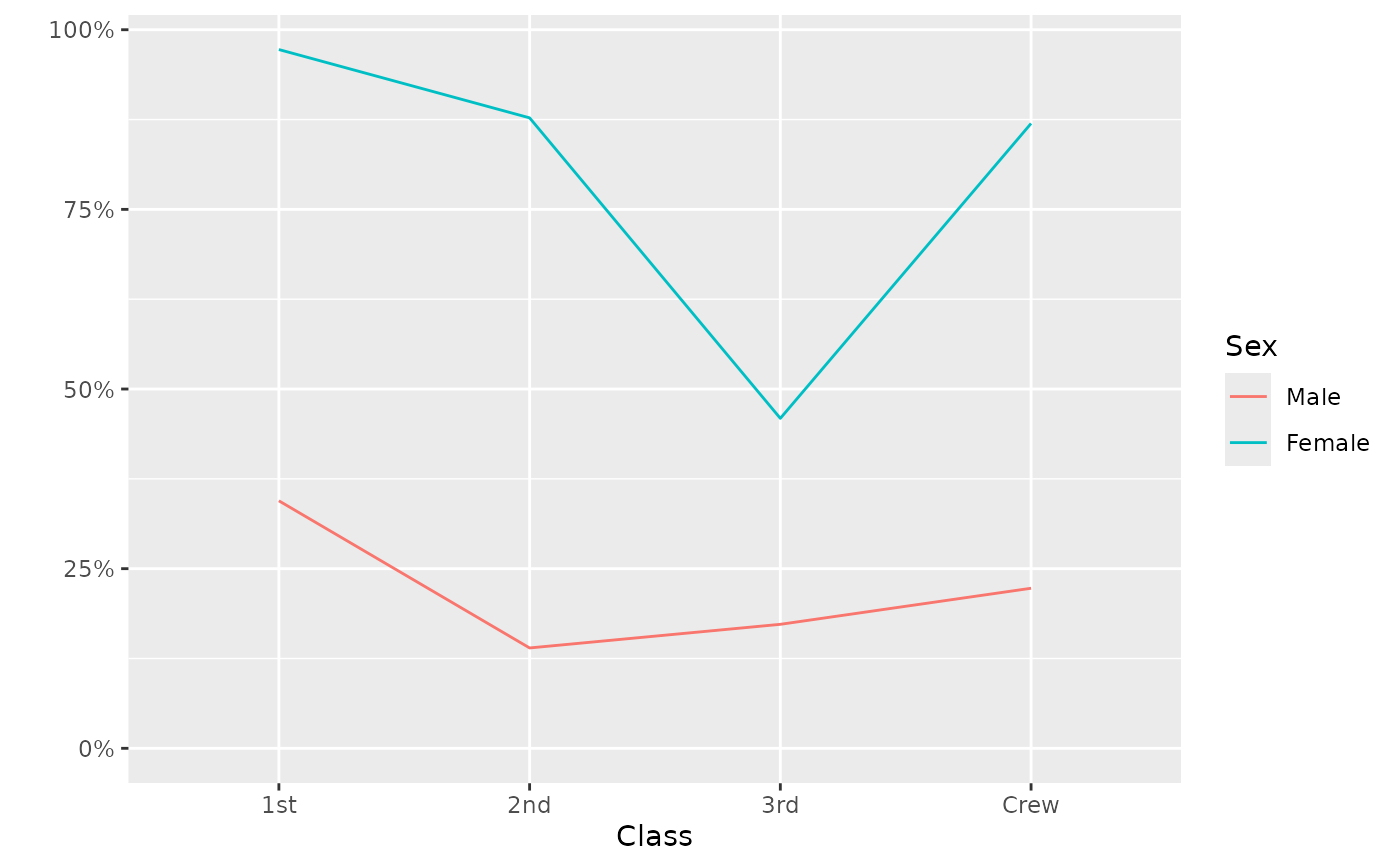Plot trends using line plots. For continuous y variables, plot the evolution of the mean. For binary y variables, plot the evolution of the proportion.

ggally_trends(data, mapping, ..., include_zero = FALSE)

## Arguments

data data set using aesthetics being used other arguments passed to ggplot2::geom_line() Should 0 be included on the y-axis?

## Author

Joseph Larmarange

## Examples

# Small function to display plots only if it's interactive
p_ <- GGally::print_if_interactive

data(tips, package = "reshape")
tips_f <- tips
tips_f$day <- factor(tips$day, c("Thur", "Fri", "Sat", "Sun"))

# Numeric variable
p_(ggally_trends(tips_f, mapping = aes(x = day, y = total_bill)))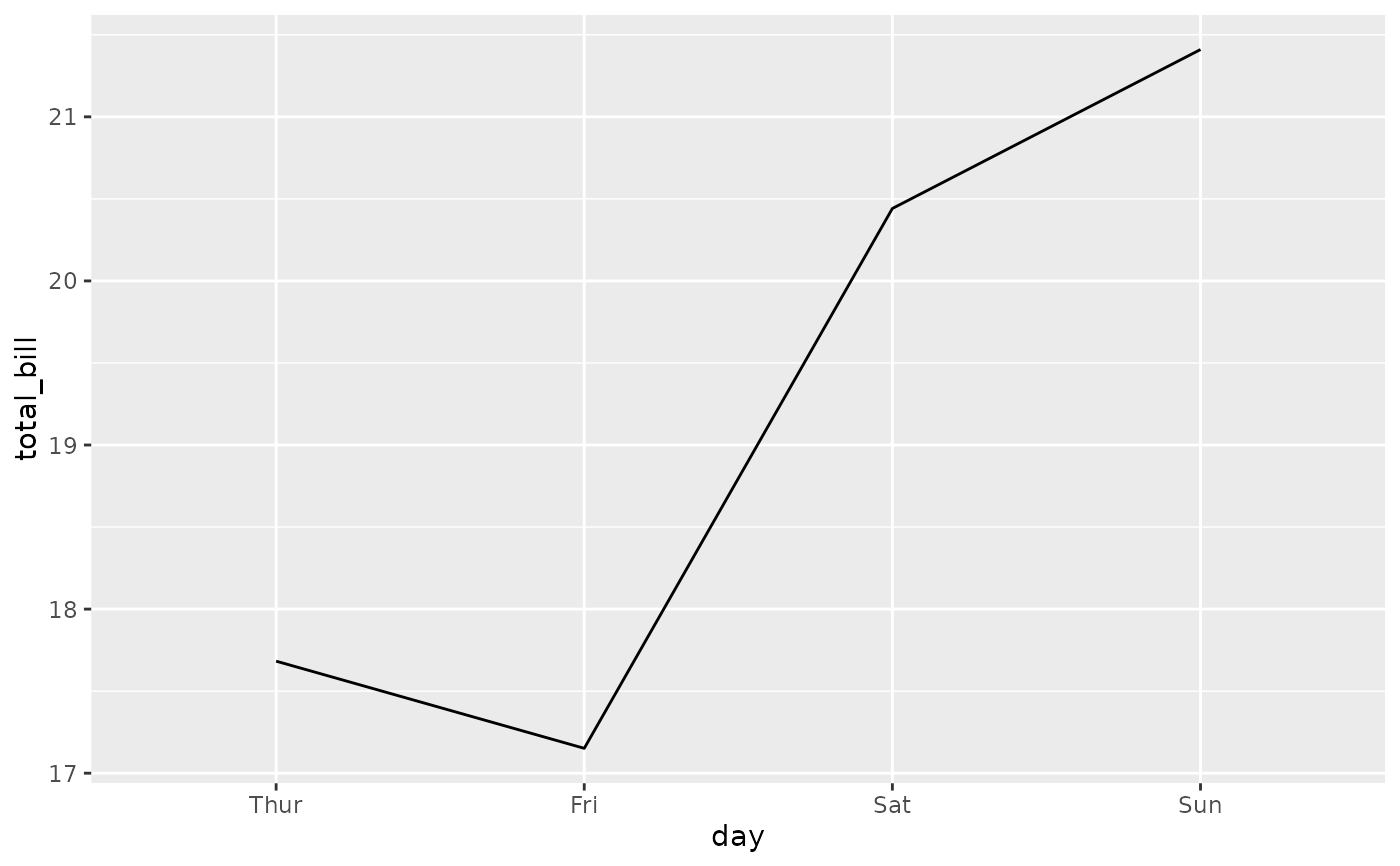p_(ggally_trends(tips_f, mapping = aes(x = day, y = total_bill, colour = time)))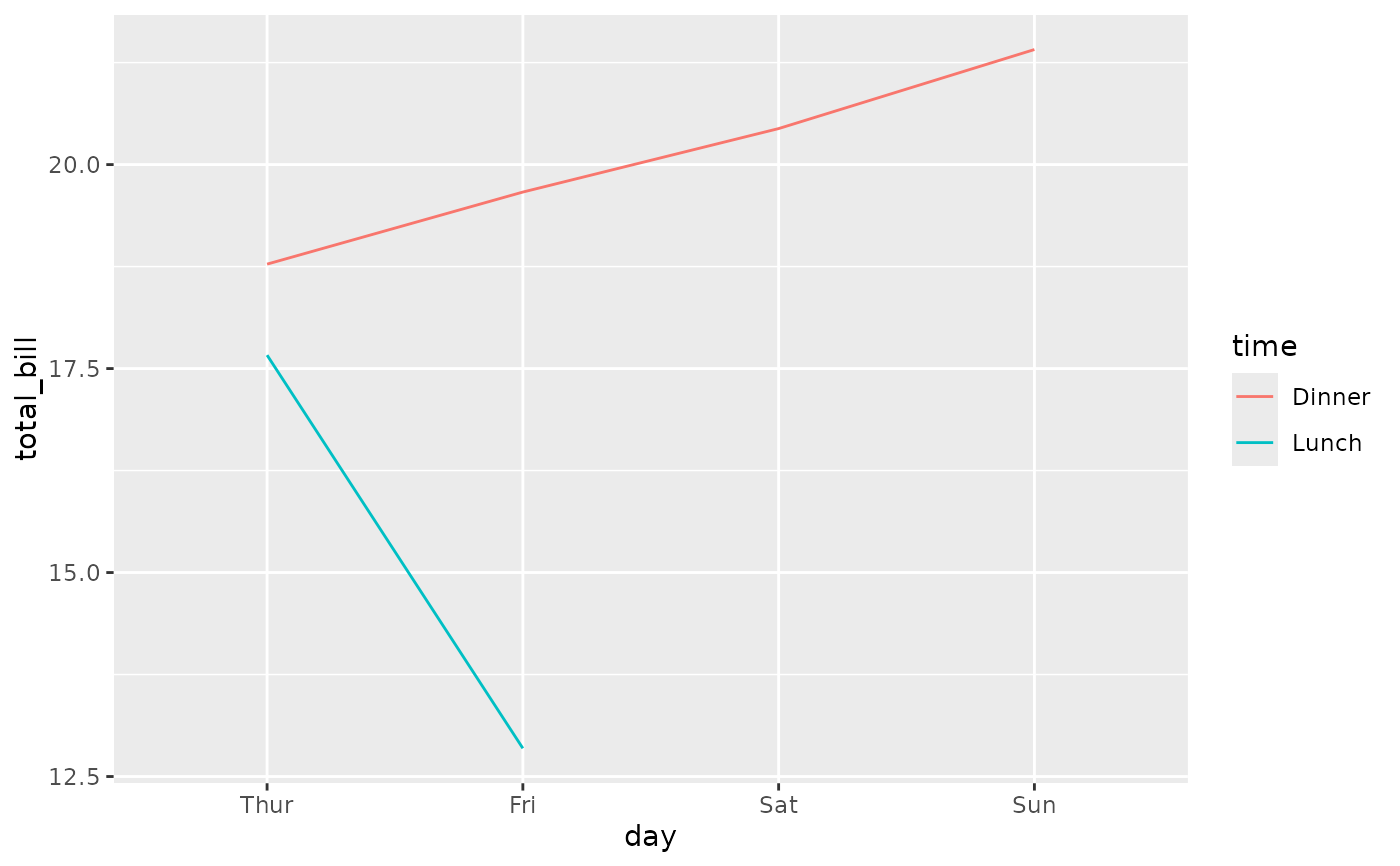# Binary variable
p_(ggally_trends(tips_f, mapping = aes(x = day, y = smoker)))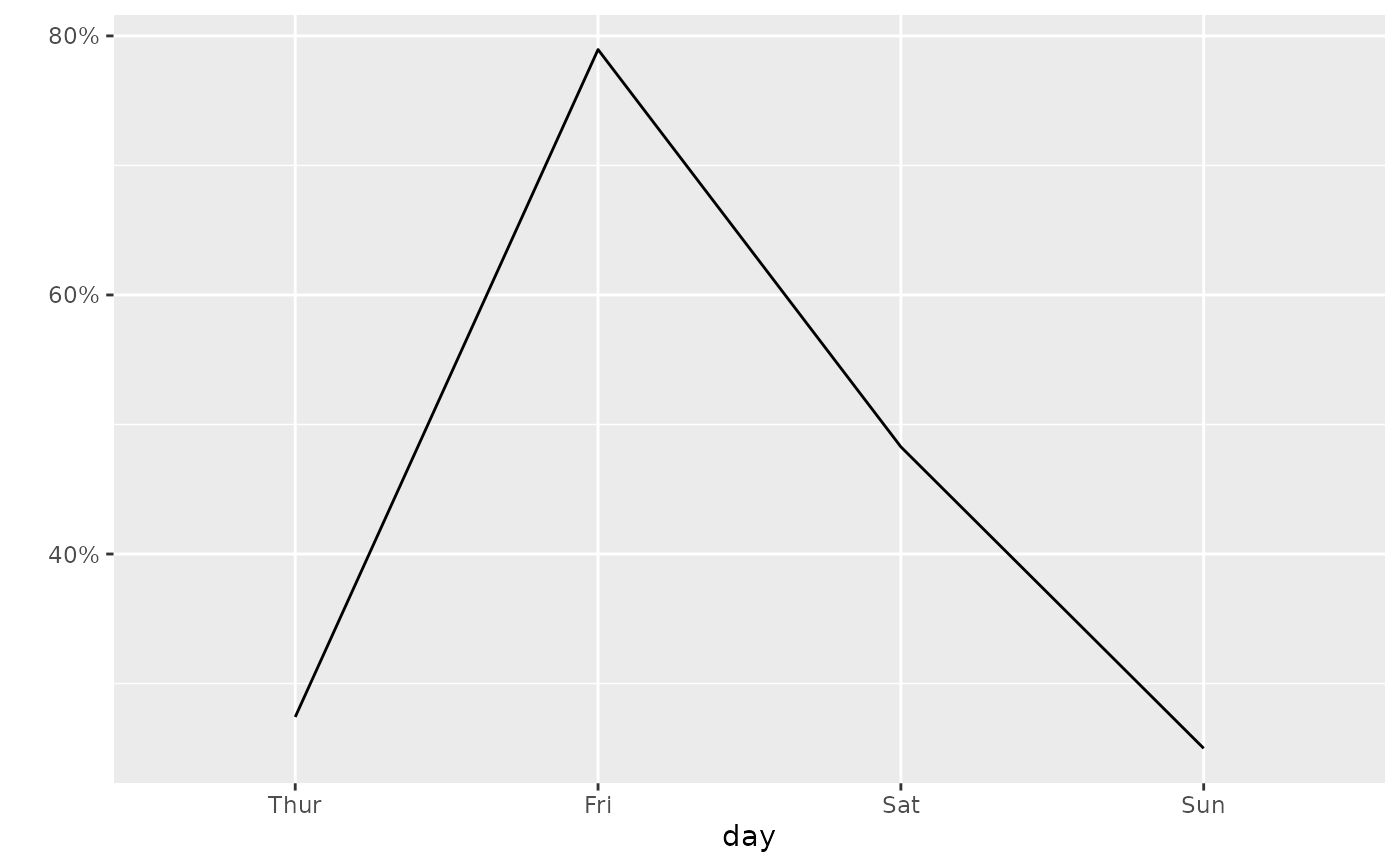p_(ggally_trends(tips_f, mapping = aes(x = day, y = smoker, colour = sex)))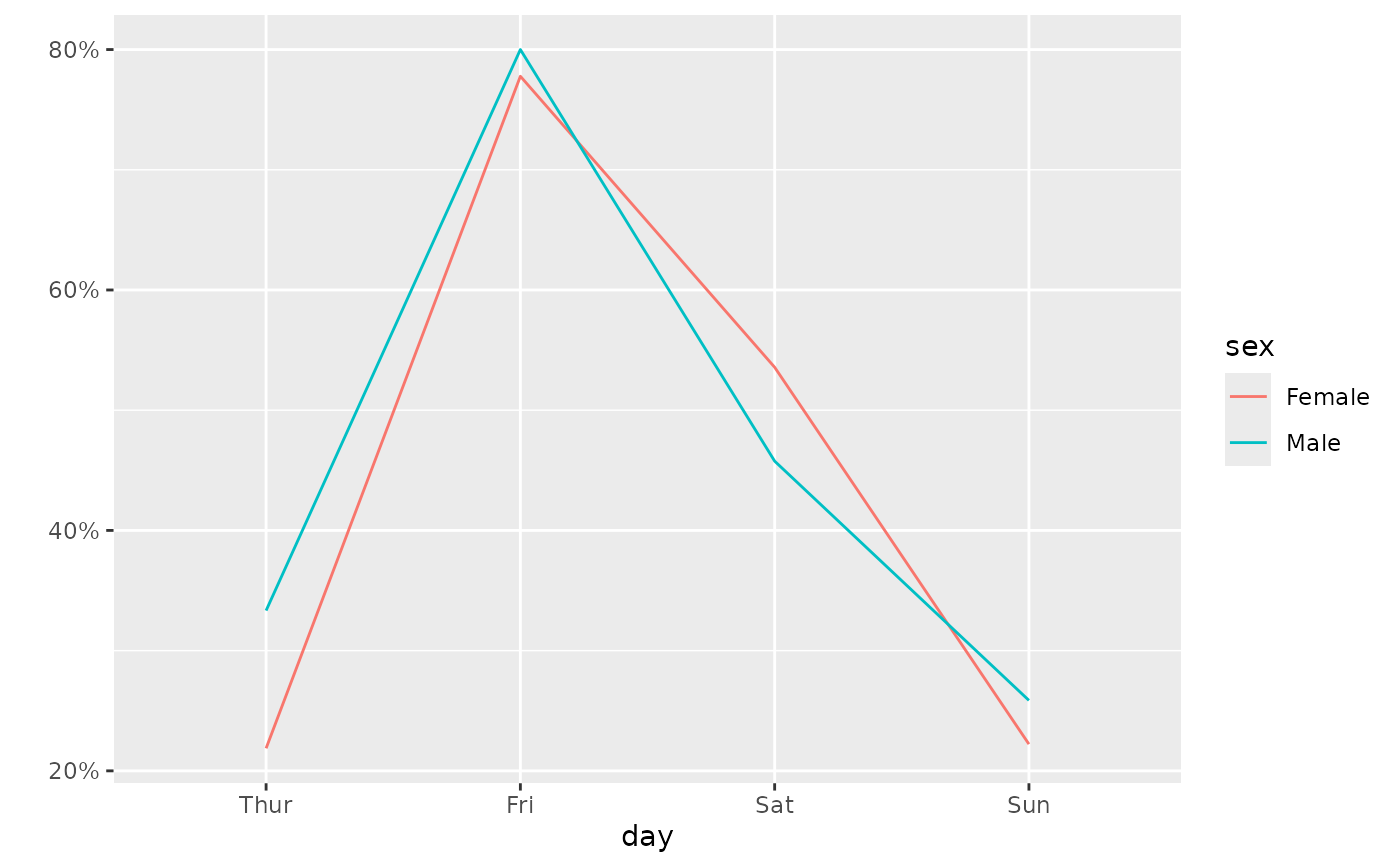# Discrete variable with 3 or more categories
p_(ggally_trends(tips_f, mapping = aes(x = smoker, y = day)))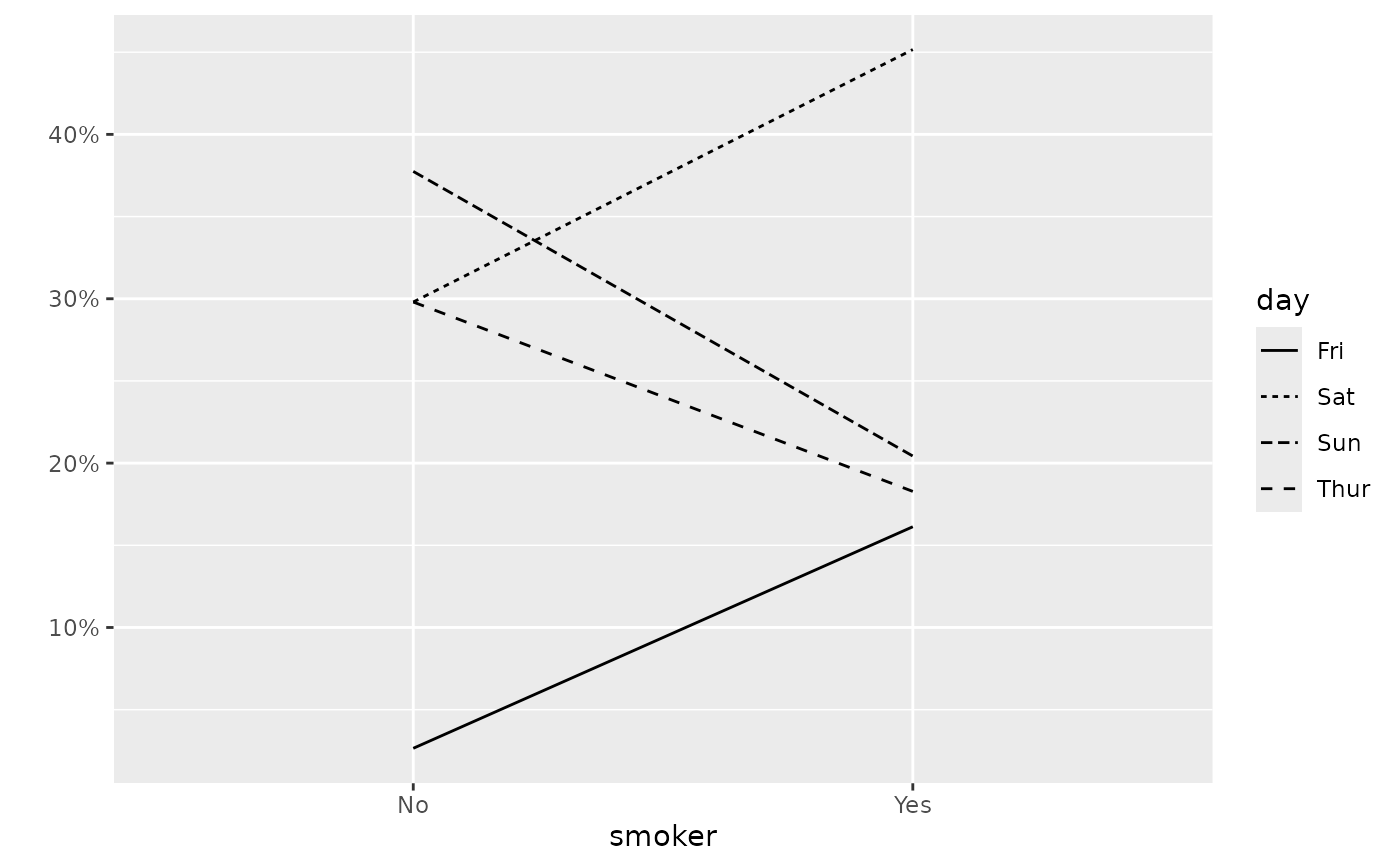p_(ggally_trends(tips_f, mapping = aes(x = smoker, y = day, color = sex)))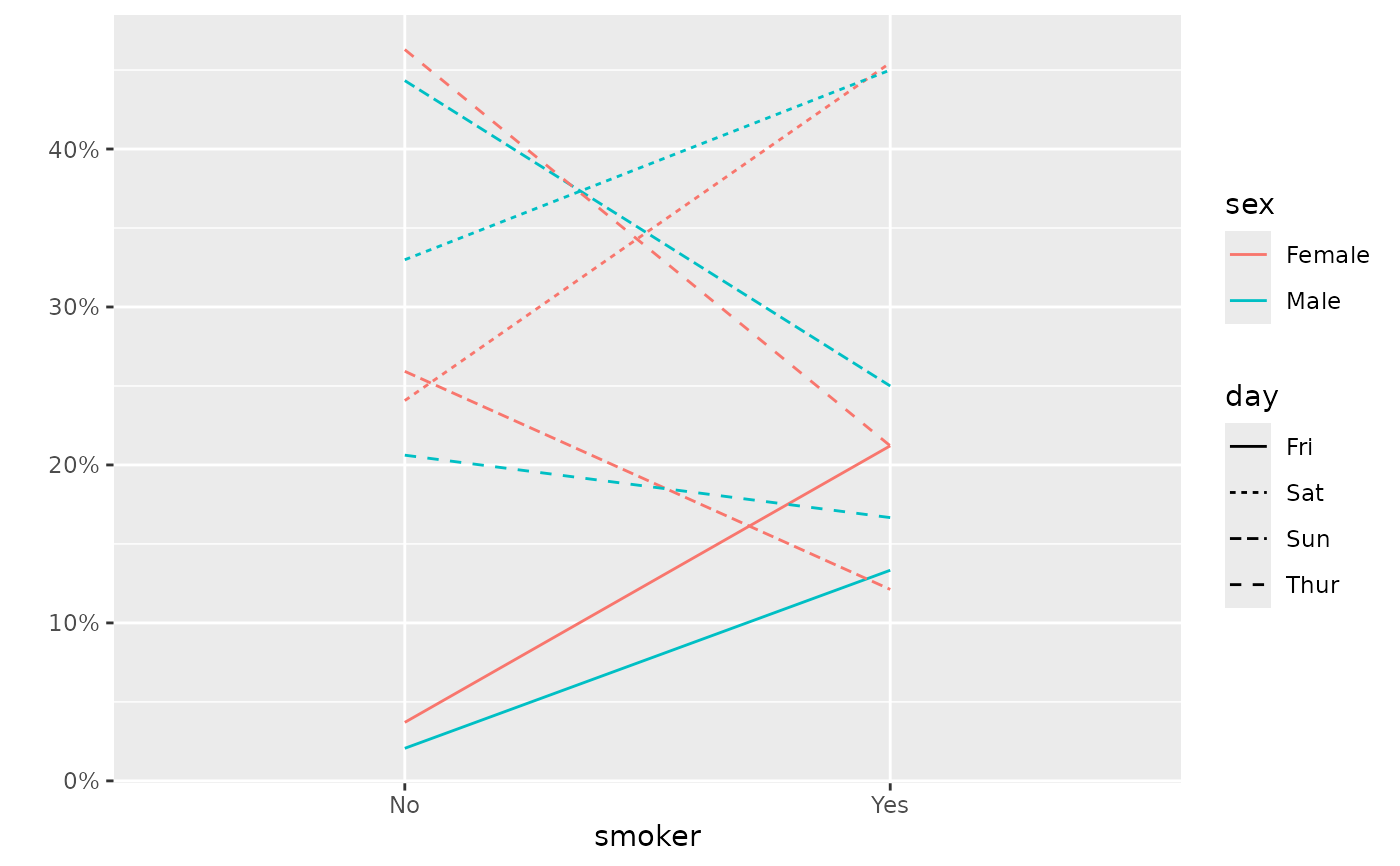# Include zero on Y axis
p_(ggally_trends(tips_f, mapping = aes(x = day, y = total_bill), include_zero = TRUE))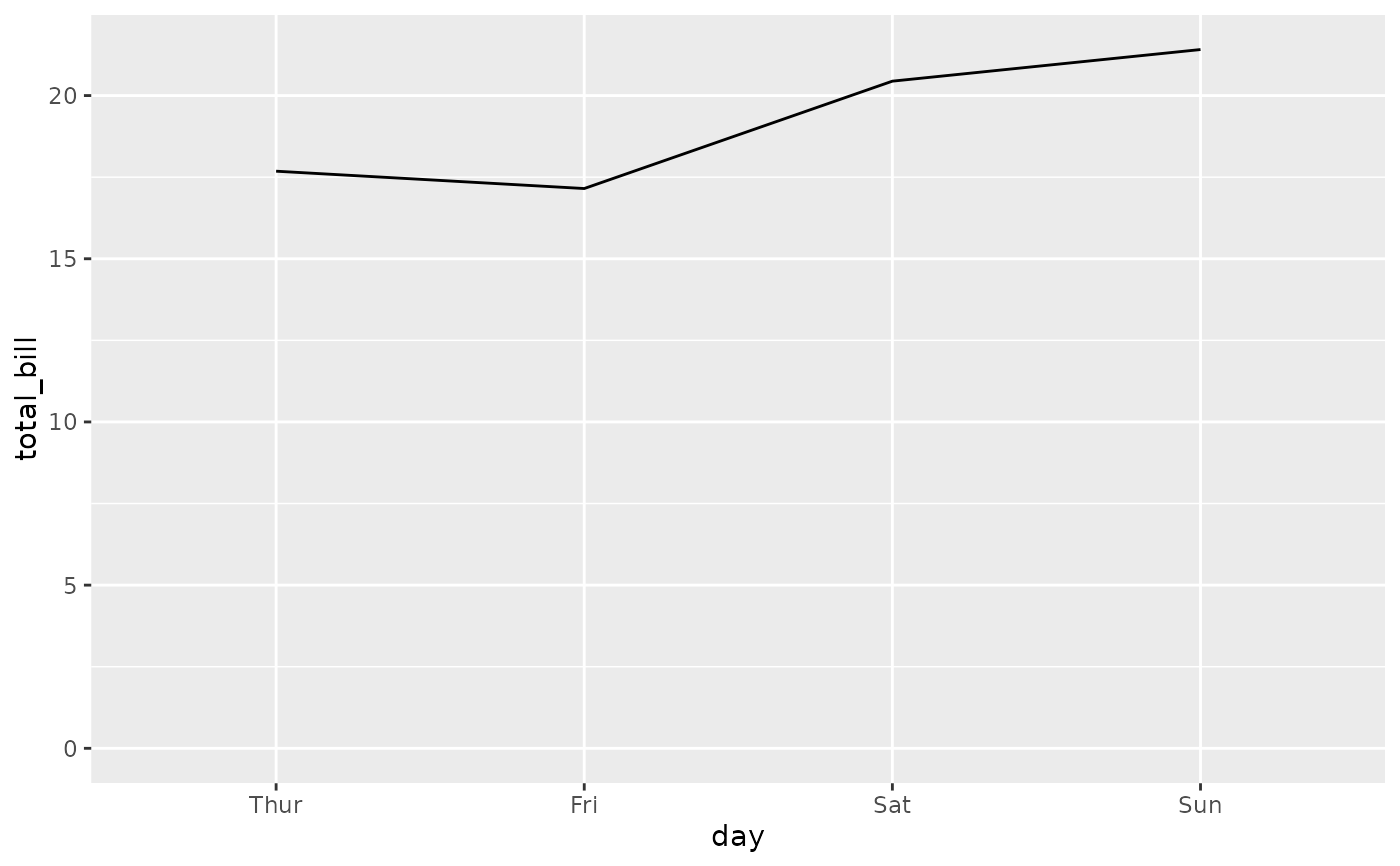p_(ggally_trends(tips_f, mapping = aes(x = day, y = smoker), include_zero = TRUE))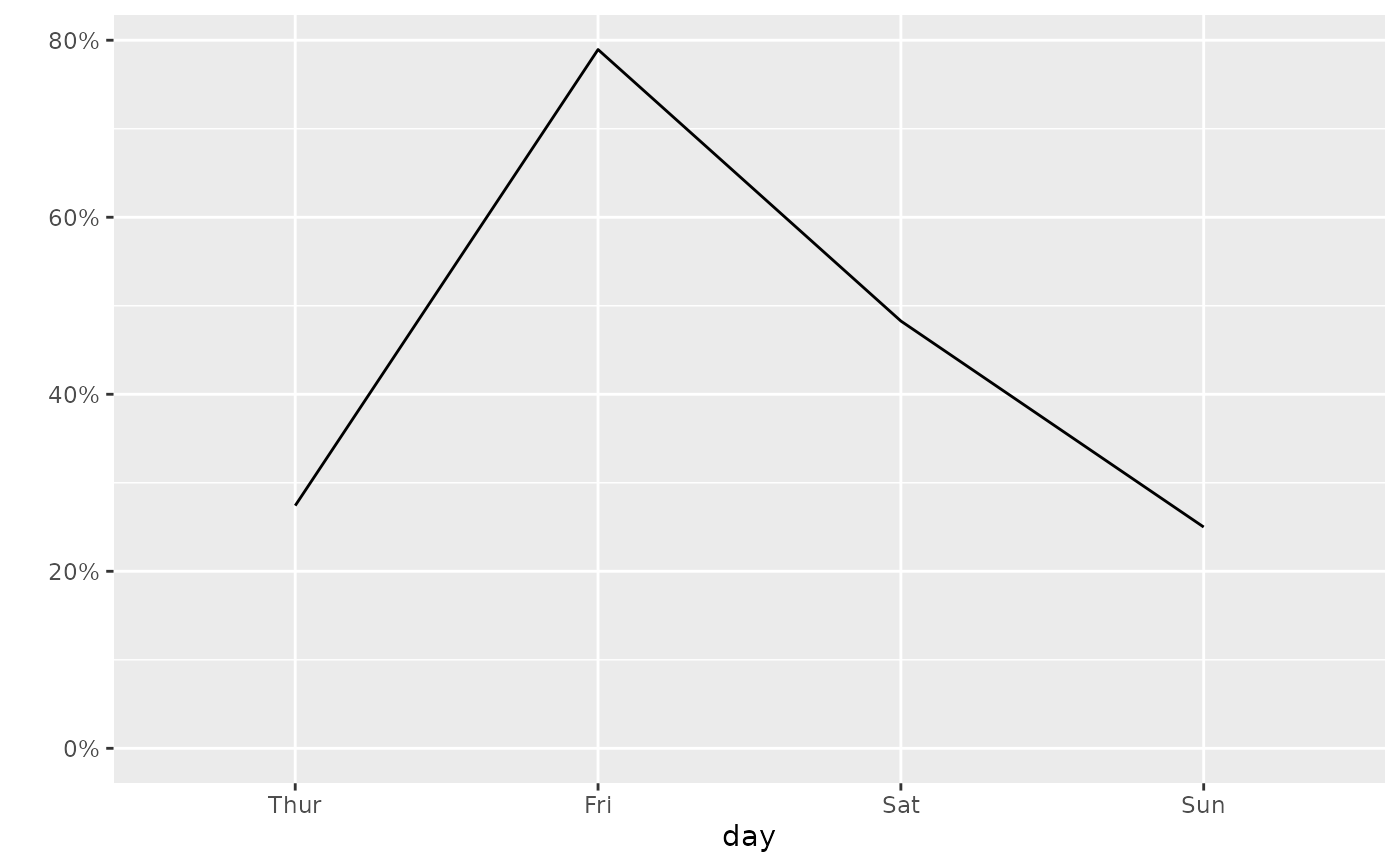# Change line size
p_(ggally_trends(tips_f, mapping = aes(x = day, y = smoker, colour = sex), size = 3))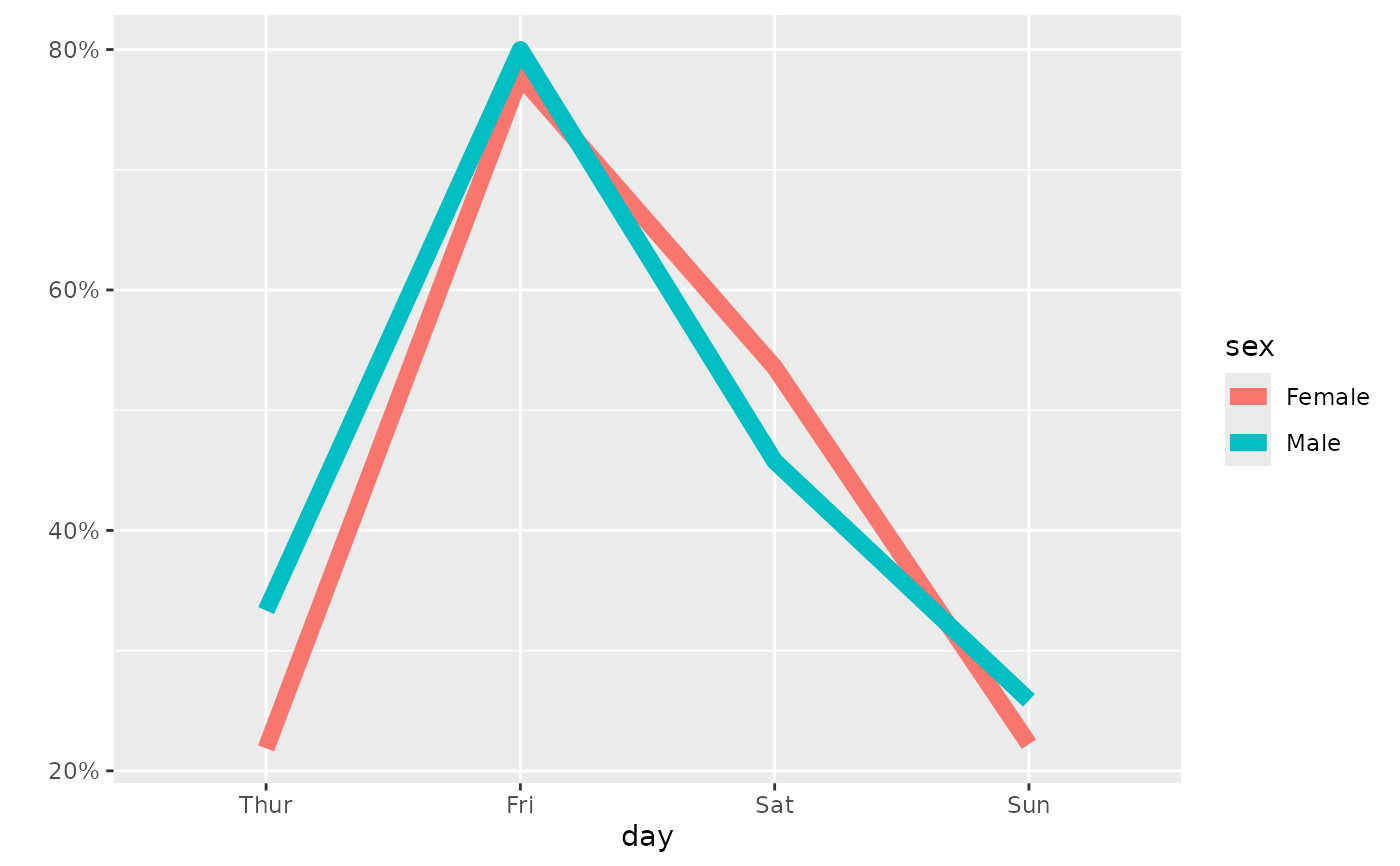# Define weights with the appropriate aesthetic
d <- as.data.frame(Titanic)
p_(ggally_trends(
d,
mapping = aes(x = Class, y = Survived, weight = Freq, color = Sex),
include_zero = TRUE
))